NEET  >  Test: Electromagnetic Induction - 1

# Test: Electromagnetic Induction - 1

Test Description

## 30 Questions MCQ Test Physics Class 12 | Test: Electromagnetic Induction - 1

Test: Electromagnetic Induction - 1 for NEET 2023 is part of Physics Class 12 preparation. The Test: Electromagnetic Induction - 1 questions and answers have been prepared according to the NEET exam syllabus.The Test: Electromagnetic Induction - 1 MCQs are made for NEET 2023 Exam. Find important definitions, questions, notes, meanings, examples, exercises, MCQs and online tests for Test: Electromagnetic Induction - 1 below.
Solutions of Test: Electromagnetic Induction - 1 questions in English are available as part of our Physics Class 12 for NEET & Test: Electromagnetic Induction - 1 solutions in Hindi for Physics Class 12 course. Download more important topics, notes, lectures and mock test series for NEET Exam by signing up for free. Attempt Test: Electromagnetic Induction - 1 | 30 questions in 30 minutes | Mock test for NEET preparation | Free important questions MCQ to study Physics Class 12 for NEET Exam | Download free PDF with solutions
 1 Crore+ students have signed up on EduRev. Have you?
Test: Electromagnetic Induction - 1 - Question 1

### electromagnetic induction i.e currents can be induced in coils (Select the best)

Detailed Solution for Test: Electromagnetic Induction - 1 - Question 1

Explanation:for electromagnetic induction magnet and coil both may move but relative motion between must be present

Test: Electromagnetic Induction - 1 - Question 2

### Damping in galvanometers is based oUpdate Questionn

Detailed Solution for Test: Electromagnetic Induction - 1 - Question 2

Explanation : The coil of galvanometer is wound on a light metal frame. When the coil and frame rotate in the field of the permanent magnet, the eddy current set up in the frame oppose the motion so that the coil returns to zero quickly.

Test: Electromagnetic Induction - 1 - Question 3

### Predict the polarity of the capacitor in the situation described by fig.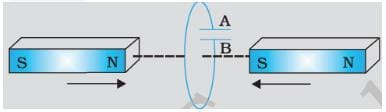Detailed Solution for Test: Electromagnetic Induction - 1 - Question 3

Explanation:A will become positive with respect to B because current  indused is in clockwise direction

Test: Electromagnetic Induction - 1 - Question 4

A circular coil of radius 8.0 cm and 20 turns is rotated about its vertical diameter with an angular speed of 50 rad s-1 in a uniform horizontal magnetic field of magnitude 3×10-2 T. If the coil resistance is 10Ω, maximum emf induced in the coil,maximum value of current in the coil and average power loss due to Joule heating are

Detailed Solution for Test: Electromagnetic Induction - 1 - Question 4

e = NBAω = 20 x 3 x 10-2 x (3.14 x 0.08 x 0.08) x 50 = 0.603C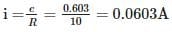average power loss due to joule heating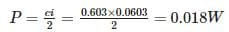Test: Electromagnetic Induction - 1 - Question 5

An ac generator consists of 8 turns of wire, each of area A=0.0900m2 , and the total resistance of the wire is 12.0Ω. The loop rotates in a 0.500-T magnetic field at a constant frequency of 60.0 Hz. Maximum induced emf is

Detailed Solution for Test: Electromagnetic Induction - 1 - Question 5

e = NBAω = NBA2πn = 8 x 0.5 x 0.09 x 2 x 3.14 x 60 = 136V

Test: Electromagnetic Induction - 1 - Question 6

When a bar magnet is pushed towards the coil connected in series with a galvanometer, the pointer in the galvanometer G

Detailed Solution for Test: Electromagnetic Induction - 1 - Question 6

Explanation:When  bar is in motion then flux will changed hence current will be induced.

Test: Electromagnetic Induction - 1 - Question 7

There are two stationary coils near each other. If current in coil-1 is changing with time, relationship of current in coil-2 to it's emf is described by

Detailed Solution for Test: Electromagnetic Induction - 1 - Question 7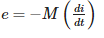M is mutual inductance

Test: Electromagnetic Induction - 1 - Question 8

A metallic rod of 1 m length is rotated with a frequency of 50 rev/s about an axis passing through the centre point O. Other end of the metallic rod slides on a Metallic ring . A constant and uniform magnetic field of 2 T parallel to the axis is present everywhere. What is the emf between the centre and the metallic ring?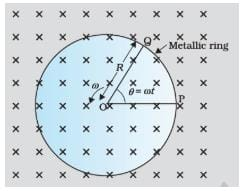Detailed Solution for Test: Electromagnetic Induction - 1 - Question 8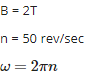l = 1 m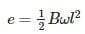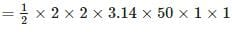= 314V

Test: Electromagnetic Induction - 1 - Question 9

Coils C1 and C2 are stationary. C1 connected in series with a galvanometer while C2 is connected to a battery through a tapping key. If the tapping key is pressed and released the galvaometer

Detailed Solution for Test: Electromagnetic Induction - 1 - Question 9

Explanation:flux will be changed when current in C2 is changing. Hence current will be induced in coil C1.

Test: Electromagnetic Induction - 1 - Question 10

An AC generator consists of

Detailed Solution for Test: Electromagnetic Induction - 1 - Question 10

Explanation:AC generators  operate on the same fundamental principles of electromagnetic induction.The turning of a coil in a magnetic field produces motional emfs in both sides of the coil.A slip ring  is a method of making an electrical connection through a rotating assembly. Formally, it is an electric transmission device that allows energy flow between two electrical rotating parts, such as in a motor.

Test: Electromagnetic Induction - 1 - Question 11

Detailed Solution for Test: Electromagnetic Induction - 1 - Question 11

Explanation:The induced electromotive force in any closed circuit is equal to the negative of the time rate of change of the magnetic flux enclosed by the circuit.

Test: Electromagnetic Induction - 1 - Question 12

Current in a circuit falls from 5.0 A to 0.0 A in 0.1 s. If an average emf of 200 V induced, estimate of the self-inductance of the circuit is

Detailed Solution for Test: Electromagnetic Induction - 1 - Question 12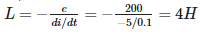Test: Electromagnetic Induction - 1 - Question 13

The magnetic field between the Horizontal poles of an electromagnet is uniform at any time, but its magnitude is increasing at the rate of 0.020T/s.The area of a horizontal conducting loop in the magnetic field is 120cm2, and the total circuit resistance, including the meter, is 5Ω. Induced emf and the induced current in the circuit are

Detailed Solution for Test: Electromagnetic Induction - 1 - Question 13

Explanation:

e = Rate of change of magnetic field x area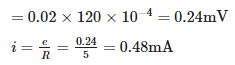Test: Electromagnetic Induction - 1 - Question 14

Coils C1 and C2 are stationary. C1 connected in series with one very small diwali bulb while C2 is connected to a battery .If the connection is suddenly snapped

Detailed Solution for Test: Electromagnetic Induction - 1 - Question 14

Explanation:Flux will be changed due to this current is induced momentarily

Test: Electromagnetic Induction - 1 - Question 15

If AC generator coil has area A, rotates in a magnetic field of B with an angular speed ω , the voltage generated is

Detailed Solution for Test: Electromagnetic Induction - 1 - Question 15

Explanation:e=NBAωSinωt

Test: Electromagnetic Induction - 1 - Question 16

Kamla peddles a stationary bicycle the pedals of the bicycle are attached to a 100 turn coil of area 0.10m2. The coil rotates at half a revolution per second and it is placed in a uniform magnetic field of 0.02 T perpendicular to the axis of rotation of the coil. What is the maximum voltage generated in the coil?

Detailed Solution for Test: Electromagnetic Induction - 1 - Question 16

Maximum voltage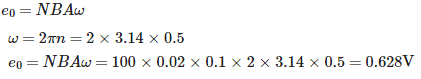Test: Electromagnetic Induction - 1 - Question 17

A pair of adjacent coils has a mutual inductance of 1.5 H. If the current in one coil changes from 0 to 20 A in 0.5 s, change of flux linkage with the other coil is

Detailed Solution for Test: Electromagnetic Induction - 1 - Question 17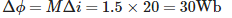Test: Electromagnetic Induction - 1 - Question 18

The magnetic field between the Horizontal poles of an electromagnet in is uniform at any time, but its magnitude is increasing at the rate of 0.020 T/s.The area of a loop made of perfect insulating material is 120cm2.This loop is kept horizontally in the magnetic field. Induced emf and the induced current in the circuit are

Detailed Solution for Test: Electromagnetic Induction - 1 - Question 18

e = Rate of change of magnetic field x area

=0.02×120×10−4=0.24mV

Test: Electromagnetic Induction - 1 - Question 19

If the polarity of induced emf is such that it tends to produce a current which aids the change in magnetic flux that produced it, which conservation law is violated?

Detailed Solution for Test: Electromagnetic Induction - 1 - Question 19

Explanation : In this case we would be obtaining electrical energy continuously without doing any work.

Test: Electromagnetic Induction - 1 - Question 20

A rectangular loop and a circular loop are moving out of a uniform magnetic field region to a field-free region with a constant velocity v as shown in fig. In which loop do you except  the induced EMF to be constant during the passage out of the field region if the field is normal to the loops.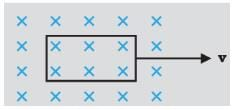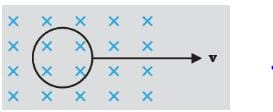Detailed Solution for Test: Electromagnetic Induction - 1 - Question 20

Explanation:The induced EMF is expected to be constant in the case of a rectangular loop. In case of a circular loop, the rate of change of area of the loop during the passage out of the field region is not constant, whereas it is constant for rectangular loop.

Test: Electromagnetic Induction - 1 - Question 21

A rectangular conductor PQRS in which the conductor PQ is free to move is kept in a magnetic field B ,which is perpendicular to the PQRS which lies in a single plane. If the conductor PQ has a length l,moves with a speed v, then induced emf is

Detailed Solution for Test: Electromagnetic Induction - 1 - Question 21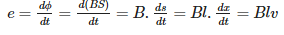Test: Electromagnetic Induction - 1 - Question 22

A 500-loop circular wire coil with radius 4.00 cm is placed between the poles of a large electromagnet. The magnetic field is uniform and makes an angle of 60 with the plane of the coil; it decreases at 0.200 T/s . Magnitude of induced emf is

Detailed Solution for Test: Electromagnetic Induction - 1 - Question 22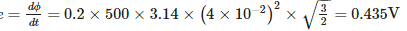Test: Electromagnetic Induction - 1 - Question 23

Use Lenz’s law to determine the direction of induced current in the situations described by the Figure (a) A wire of irregular shape turning into a circular shape; (b) A circular loop being deformed into a narrow straight wire. The directions for (a) and (b) respectively are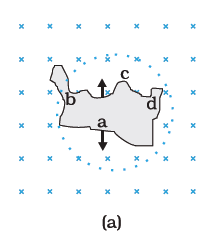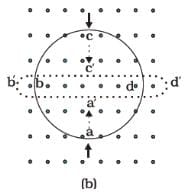Detailed Solution for Test: Electromagnetic Induction - 1 - Question 23

Explanation:(a) for a given Periphery the area of a circle is maximum. When a coil takes a circular shape, the magnetic flux linked with the coil increases, so the current induced in the coil will tend to decrease the flux and so it will produce a magnetic field upward. As a result the current induced in the coil will flow in anticlockwise direction.(b) When circular coil takes the shape of narrow straight wire, The magnetic flux linked with the coil decreases. So the current induced in the coil will tend to oppose the decrease in magnetic flux. Hence it will produce an upward magnetic field so that the current induced in the coil will flow in anticlockwise direction.

Test: Electromagnetic Induction - 1 - Question 24

An air-cored solenoid with length 30 cm, area of cross-section 25 cm2 and number of turns 500, carries a current of 2.5 A. The current is suddenly switched off in a brief time of 10−3s. Average back emf induced across the ends of the open switch in the circuit is

Detailed Solution for Test: Electromagnetic Induction - 1 - Question 24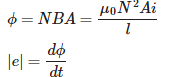Test: Electromagnetic Induction - 1 - Question 25

If two coils of inductances L1 and L2 are linked such that their mutual inductance is M, then

Detailed Solution for Test: Electromagnetic Induction - 1 - Question 25

M=k√L1L2

here k is cofficient of coupling. Its maximum value is 1 for tight coupling.

Test: Electromagnetic Induction - 1 - Question 26

Eddy Currents are

Detailed Solution for Test: Electromagnetic Induction - 1 - Question 26

Explanation:Eddy currents  (also called Foucault currents) are loops of electrical current induced within conductors by a changing magnetic field in the conductor, due to Faraday's law of induction. Eddy currents flow in closed loops within conductors, in planes perpendicular to the magnetic field. By lenz's law an eddy current creates a magnetic field that opposes a change in the magnetic field that created it, and thus eddy currents react back on the source of the magnetic field.

Test: Electromagnetic Induction - 1 - Question 27

Polarity of the capacitor in the situation described by the adjacent figure is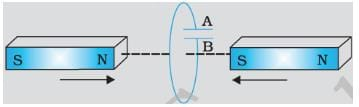Detailed Solution for Test: Electromagnetic Induction - 1 - Question 27

Explanation:A will become positive with respect to B because current  indused is in clockwise direction

Test: Electromagnetic Induction - 1 - Question 28

A 1.0 m long metallic rod is rotated with an angular frequency of 400 rad s−1 about an axis normal to the rod passing through its one end. The other end of the rod is in contact with a circular metallic ring. A constant and uniform magnetic field of 0.5 T parallel to the axis exists everywhere. Emf developed between the centre and the ring is

Detailed Solution for Test: Electromagnetic Induction - 1 - Question 28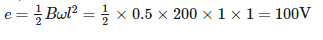Test: Electromagnetic Induction - 1 - Question 29

On a cylindrical rod two coils are wound one above the other. What is the coefficient of mutual inductance if the inductance of each coil os 0.1H?

Detailed Solution for Test: Electromagnetic Induction - 1 - Question 29

As one coil is wound over the other so that coupling is tight i.e. k=1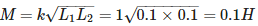Test: Electromagnetic Induction - 1 - Question 30

Two ends of a horizontal conducting rod of length l are joined to a voltmeter. The whole arrangement moves with a horizontal velocity v, the direction of motion being perpendicular to the rod. Vertical component of the earth's magnetic field is B. The voltmeter reads

Detailed Solution for Test: Electromagnetic Induction - 1 - Question 30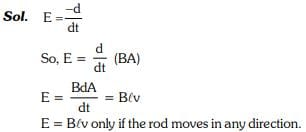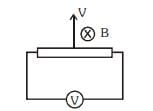## Physics Class 12

157 videos|452 docs|213 tests
 Use Code STAYHOME200 and get INR 200 additional OFF Use Coupon Code
Information about Test: Electromagnetic Induction - 1 Page
In this test you can find the Exam questions for Test: Electromagnetic Induction - 1 solved & explained in the simplest way possible. Besides giving Questions and answers for Test: Electromagnetic Induction - 1, EduRev gives you an ample number of Online tests for practice

## Physics Class 12

157 videos|452 docs|213 tests

### How to Prepare for NEET

Read our guide to prepare for NEET which is created by Toppers & the best Teachers